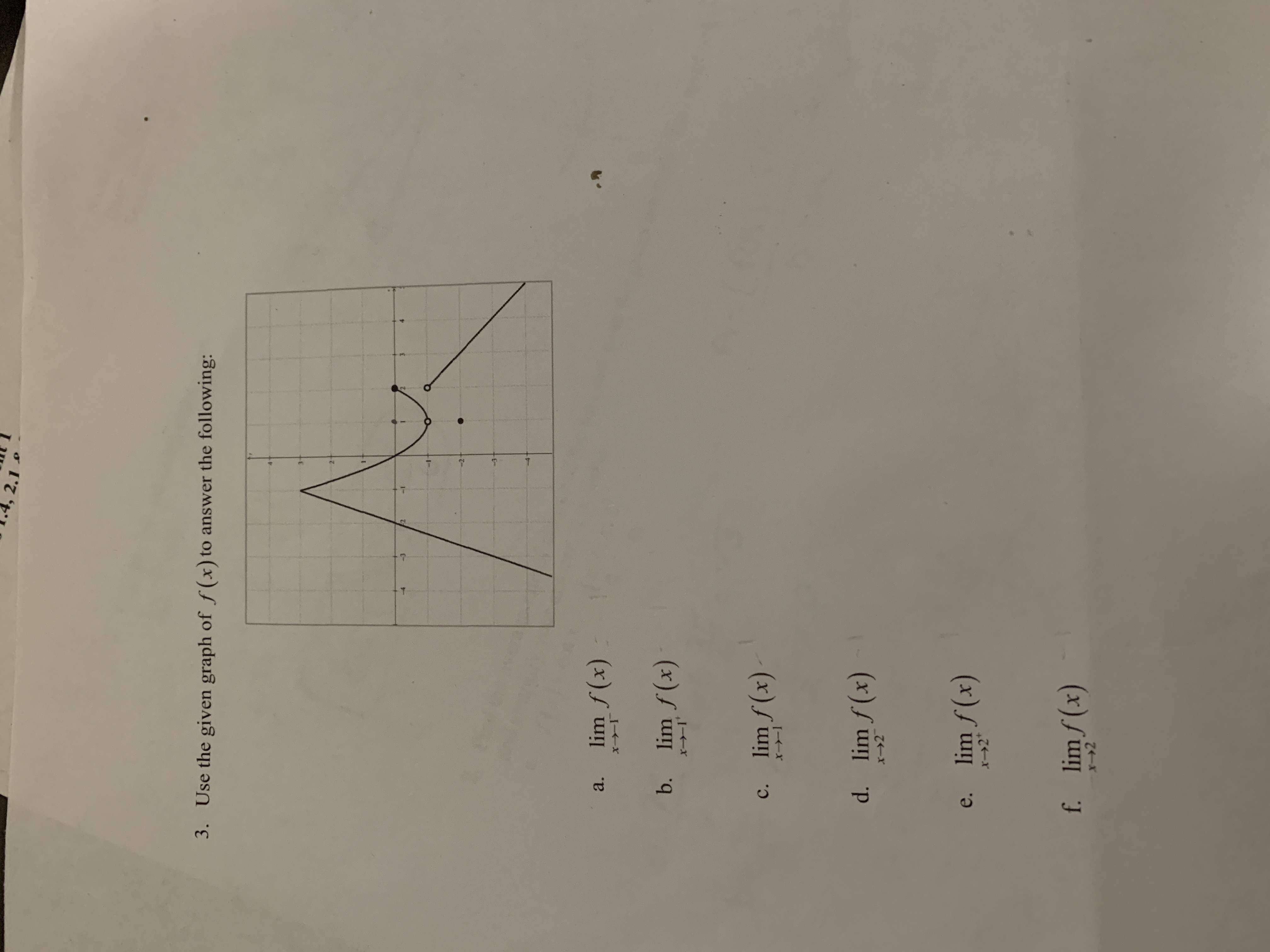# 2.1 Use the given graph of f (x) to answer the following: 3. 3 $2 lim f(x) a. b. lim f (x) x-1 lim f (x) с. X-1 d. lim f(x) x-2 lim f (x) e. f. limf (x) X--2 Question Use the graph of f(x) to answer the followinghelp_outlineImage Transcriptionclose2.1 Use the given graph of f (x) to answer the following: 3. 3$2 lim f(x) a. b. lim f (x) x-1 lim f (x) с. X-1 d. lim f(x) x-2 lim f (x) e. f. limf (x) X--2 fullscreen

1 Rating

### Want to see this answer and more?

Experts are waiting 24/7 to provide step-by-step solutions in as fast as 30 minutes!*

*Response times vary by subject and question complexity. Median response time is 34 minutes and may be longer for new subjects.
Tagged in
MathCalculus

### Limits